2016-11-07 16:12:12 LG1259156776 阅读数 8882
• ###### MATLAB图像处理

全面系统的学习MATLAB在图像处理中的应用

19898 人正在学习 去看看 魏伟

1.

2.

# 2. Hu矩

mpq=i=1Mj=1Nipjqf(i,j)

μpq=i=1Mj=1N(ii¯)p(jj¯)qf(i,j)

ηpq=μpaμγ00,(γ=p+q2,p+q=2,3,)

# 3. 利用OpenCV计算Hu矩

opencv里对Hu矩的计算有直接的API，它分为了两个函数：moments()函数用于计算中心矩，HuMoments函数用于由中心矩计算Hu矩。

Moments moments(InputArray array, bool binaryImage=false )

## 参数说明

• 输入参数：array是一幅单通道，8-bits的图像，或一个二维浮点数组(Point of Point2f)。binaryImage用来指示输出图像是否为一幅二值图像，如果是二值图像，则图像中所有非0像素看作为1进行计算。
• 输出参数：moments是一个类：
class Moments
{
public:
Moments();
Moments(double m00, double m10, double m01, double m20, double m11,
double m02, double m30, double m21, double m12, double m03 );
Moments( const CvMoments& moments );
operator CvMoments() const;

void HuMoments(const Moments& moments, double* hu)

## 参数说明：

• 输入参数：moments即为上面一个函数计算得到的moments类型。
• 输出参数：hu是一个含有7个数的数组。

int main(int argc, char** argv)

cvtColor(image, image, CV_BGR2GRAY);
Moments mts = moments(image);
double hu;
HuMoments(mts, hu);
for (int i=0; i<7; i++)
{
cout << log(abs(hu[i])) <<endl;
}
return 0;
}

 类别 原图 -6.76181 -19.1286 -23.7441 -26.776 -51.7618 -35.8491 -51.534 旋转 -6.72102 -19.0844 -23.5756 -25.9122 -51.4619 -35.4595 -50.7674 加放噪点 -6.76086 -19.1255 -23.7611 -26.3228 -51.5056 -35.895 -51.6321 模糊 -6.76183 -19.1295 -23.7451 -26.2767 -51.765 -35.8484 -51.5307

# 4. Zernike矩

Hu矩在图像描述上有广泛的应用，但是其低阶几何矩与图像整体特征有关，不包含太多的图像细节信息，而高阶几何矩易受噪声影响，因此很难利用几何矩恢复图像。

Zernike矩能够很容易地构造图像的任意高阶矩，并能够使用较少的矩来重建图像。Zernike矩是基于Zernike多项式的正交化函数，虽然其计算比较复杂，但是Zernide矩在图像旋转和低噪声敏感度方面具有较大的优越性。由于Zernike矩具有图像旋转不变性，而且可以构造任意高阶矩，所以被广泛应用对目标进行识别中。

## 4.1 Zernike矩多项式

Zernike在1934年提出了在单位圆上定义的一组正交多项式，即Zernike正交多项式，其定义形式为：

Rnm(ρ)=s=0(n|m|)/2(1)s[(ns)!]ρn2ss!(n+|m|2s)!(n+|m|2+s)!

Vnm(x,y)=Vnm(ρ,θ)=Rnm(ρ)ejmθ

Zernike多项式

Rnm(ρ)=[(K22ρ2+K3)R(n2)m(ρ)+K4R(n4)m(ρ)]K1

Rmm(ρ)=ρm

## 4.2 Zernike矩的定义

f(x,y)=n=0m=0ZnmVn,m(ρ,θ)

Znm=n+1π102π0[Vnm(ρ,θ)]f(ρ,θ)ρdydxdρdθ

=n+1πRnm(ρ)ejmθf(ρ,θ)dρdθ

## 4.3 Zernike矩的计算

Cnm=2n+2π102π0[Rnm(ρ)cos(mθ)f(ρ,θ)ρdρdθ

Cnm=2n+2π102π0[Rnm(ρ)sin(mθ)f(ρ,θ)ρdρdθ

σ=2(rx)y|y|+xyr

σ=2yxyr

ρ=2r/N,θ=πσ(4r)

Cnm=2n+2N2r=1N/2Rnm(2r/N)σ=18rcosπmσ4rf(r,σ)

Snm=2n+2N2r=1N/2Rnm(2r/N)σ=18rsinπmσ4rf(r,σ)

1. 确定图像的大小

2. 确定

3. 利用Zernike多项式的递推性质计算各阶

4. 对

 类别 原图 11.1732 13.8469 12.3515 12.4391 14.2782 12.6137 11.5745 旋转 12.3036 13.8309 13.5861 12.0467 13.1320 13.8396 12.7862 加放噪点 11.1538 13.8490 12.3315 12.4316 14.2730 12.5925 11.5591 模糊 11.1636 13.8465 12.3480 12.4367 14.2799 12.6130 11.5752View Code

# 5. 总结

1. 选择合适的不变矩类型；
2. 选择分类器（如神经网络、最短距离等）；
3. 如果是神经网络分类器，则需要计算学习样例的不变矩去训练神经网络；
4. 计算待识别对象的不变矩，输入神经网络就可得到待识别对象的类型，或者计算待识别对象不变矩与类别对象不变矩之间的距离，选择最短距离的类别作为待识别对象的类别。

# 6. 参考资料

 《现代数字图像处理》（matlab版）

 正交多项式WIKI

 opencv形态描述

2018-12-10 10:00:49 yql_617540298 阅读数 240
• ###### MATLAB图像处理

全面系统的学习MATLAB在图像处理中的应用

19898 人正在学习 去看看 魏伟

# 一、图像

## 通过二阶矩计算图像中心：python

import imutils
import cv2
import os

#ap = argparse.ArgumentParser()
#--image 参数: 磁盘中待处理图像的路径
#args = vars(ap.parse_args())

count = os.listdir(filepath)
for i in range(0,len(count)):
print("i=",i)
gray = cv2.cvtColor(image,cv2.COLOR_BGR2GRAY)
#5×5 内核的高斯平滑
blurred = cv2.GaussianBlur(gray,(5,5),0)
#阈值化
thresh = cv2.threshold(blurred,60,255,cv2.THRESH_BINARY)

#使用轮廓检测去定位这些白色区域
#返回图像上每一个白块对应的边界点集合（即轮廓）
cnts = cv2.findContours(thresh.copy(),cv2.RETR_EXTERNAL,cv2.CHAIN_APPROX_SIMPLE)
cnts = cnts if imutils.is_cv2() else cnts

#处理每一条轮廓
for c in cnts:
#计算轮廓区域图像的矩
M = cv2.moments(c)
print("M:",M)
if M["m00"]==0:
print("skip!")
else:
#计算轮廓的中心
cX = int(M["m10"] / M["m00"])
cY = int(M["m01"] / M["m00"])
#在形状的中心 (cX, cY) 处绘制一个白色的小圆
cv2.drawContours(image, [c], -1, (0, 255, 0), 2)
cv2.circle(image, (cX, cY), 7, (255, 255, 255), -1)
cv2.putText(image, "", (cX - 20, cY - 20),
cv2.FONT_HERSHEY_SIMPLEX, 0.5, (255, 255, 255), 2)
cv2.imwrite(resultpath+str(i)+'.png',image)
#cv2.imshow("Image", image)
cv2.waitKey(0)

## 通过二阶矩计算图像重心：MATLAB

for i=0:29
bgFile = ['picture\',int2str(i),'.png'];% 读入图片的完整路径
I = rgb2gray(I);
imshow(I);
I = double(I);
[rows,cols] = size(I);
x = ones(rows,1)*[1:cols];
y = [1:rows]'*ones(1,cols);
area = sum(sum(I));
meanx = sum(sum(I.*x))/area;
meany = sum(sum(I.*y))/area;
hold on;
plot(meanx,meany,'r+'); %十字标出重心位置
F=getframe(gcf); % 获取整个窗口内容的图像
%imwrite(F.cdata,'result\1.png')
img = ['result\',num2str(i),'.png']
imwrite(F.cdata,img);
hold off;
end;

# 二、中心距求主轴方向

## 1. 中心距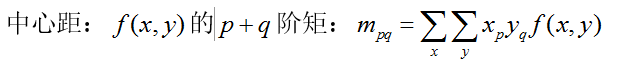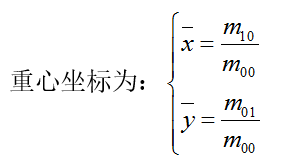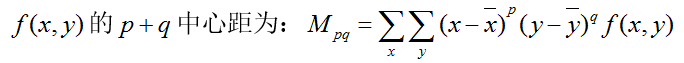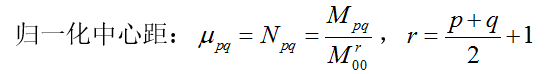## 2. 中心距求主轴方向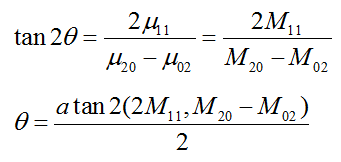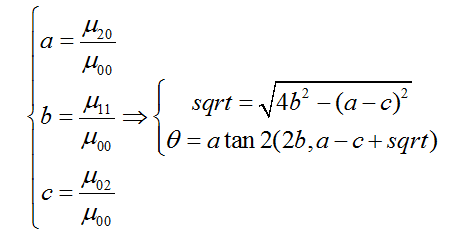## MATLAB实现：主轴方向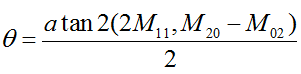function test

[cm ju] = qijieju(uint8(I));
m00 = cm(1);
mu11 = cm(2);
mu02 = cm(3);
mu20 = cm(4);
a = mu20 / m00;
b = mu11 / m00;
c = mu02 / m00;
square = sqrt( 4 * b * b + (a - c) * (a - c) );
%求主轴方法1
theta1 = atan2( 2 * b, a - c + square )*180/pi
%save('output\output.txt','theta1','-ascii')
%B = imrotate(I,theta1,'bicubic')
%imwrite(B,'output\1.png')
%求主轴方法2
theta2 = atan2(2*mu11,(mu20-mu02))/2*180/pi
I2 = imrotate(I,-theta2*10,'nearest')
imwrite(I2,'output\0.png')
end

%求不变矩及中心矩
function [cm ju] = qijieju(I0)
A=double(I0);
[nc,nr]=size(A);
[x,y]=meshgrid(1:nr,1:nc);
x=x(:);
y=y(:);
A=A(:);
m00=sum(A);
if m00==0
m00=eps;
end
m10=sum(x.*A);
m01=sum(y.*A);
xmean=m10/m00; %重心
ymean=m01/m00;
cm00=m00; %归一化中心矩
cm02=(sum((y-ymean).^2.*A))/(m00^2);
cm03=(sum((y-ymean).^3.*A))/(m00^2.5);
cm11=(sum((x-ymean).*(y-ymean).*A))/(m00^2);
cm12=(sum((x-ymean).*(y-ymean).^2.*A))/(m00^2.5);
cm20=(sum((x-xmean).^2.*A))/(m00^2);
cm21=(sum((x-xmean).^2.*(y-ymean).*A))/(m00^2.5);
cm30=(sum((x-xmean).^3.*A))/(m00^2.5);
ju(1)=cm20+cm02;  %
ju(2)=(cm20-cm02)^2+4*cm11^2; %
ju(3)=(cm30-3*cm12)^2+(3*cm21-cm03)^2; %
ju(4)=(cm30+cm12)^2+(cm21+cm03)^2;  %
ju(5)=(cm30-3*cm12)*(cm30+cm12)*((cm30+cm12)^2-3*(cm21+cm03)^2)+(3*cm21-cm03)*(cm21+cm03)*(3*(cm30+cm12)^2-(cm21+cm03)^2); %
ju(6)=(cm20-cm02)*((cm30+cm12)^2-(cm21+cm03)^2)+4*cm11*(cm30+cm12)*(cm21+cm03);  %
ju(7)=(3*cm21-cm03)*(cm30+cm12)*((cm30+cm12)^2-3*(cm21+cm03)^2)+(cm30-3*cm12)*(cm21+cm03)*(3*(cm30+cm12)^2-(cm21+cm03)^2);
qijieju= ju;%abs(log(ju))
cm = [cm00 cm11 cm02 cm20];
end

# 三、通过两点计算夹角

python实现

def azimuthAngle( x1,  y1,  x2,  y2):
angle = 0.0
dx = x2 - x1
dy = y2 - y1
angle = math.atan(dy/dx)
return (angle * 180 / math.pi)

angle = azimuthAngle(x1,y1,x2,y2)
print("angle=",angle)

2015-10-23 19:15:21 lishanlu136 阅读数 6824
• ###### MATLAB图像处理

全面系统的学习MATLAB在图像处理中的应用

19898 人正在学习 去看看 魏伟

MATLAB图像处理工具箱提供了impyramid（）函数，用于构造图像的金字塔，其调用格式如下：B=impyramid（A，direction），该函数的功能为：对A进行高斯金字塔变换，direction为'reduce'和'expand'，分别对应分解和扩张。

HU矩 几何矩是由Hu(Visual pattern recognition by moment invariants)在1962年提出的，图像f(x，y)的(p+q)阶几何矩定义为 Mpq =∫∫(x^p)*(y^q)f(x，y)dxdy(p，q = 0，1，……∞）矩在统计学中被用来反映随机变量的分布情况，推广到力学中，它被用作刻画空间物体的质量分布。同样的道理，如果我们将图像的灰度值看作是一个二维或三维的密度分布函数，那么矩方法即可用于图像分析领域并用作图像特征的提取。最常用的，物体的零阶矩表示了图像的“质量”：Moo= ∫∫f(x，y )dxdy 一阶矩(M01，M10)用于确定图像质心( Xc，Yc)：Xc = M10/M00;Yc = M01/M00;若将坐标原点移至 Xc和 Yc处，就得到了对于图像位移不变的中心矩。如Upq =∫∫[(x-Xc)^p]*[(y-Yc)^q]f(x，y)dxdy。Hu在文中提出了7个几何矩的不变量，这些不变量满足于图像平移、伸缩和旋转不变。如果定义Zpq=Upq/(U20 + U02)^(p+q+2)，Hu 的7种矩为：H1=Z20+Z02;H1=(Z20+Z02)^2+4Z11^2;......

1、运用一阶微分算子检测图像边缘

MATLAB中也提供了相关的图像边缘检测的函数，其调用格式如下：BW = edge（I,'sobel',thresh,direction)，BW = edge（I,'prewitt',thresh,direction)，

BW = edge（I,'roberts',thresh)，其中，I是输入的灰度图像，thresh是阈值，direction是方向。

2、傅里叶变换与图像梯度的关系

3、运用二阶微分算子检测图像边缘

x方向二阶微分算子=I（i，j+1）-2I（i，j）+I（i，j-1)，y方向二阶微分算子=I（i+1，j）-2I（i，j）+I（i-1，j)

4、基于Canny算子检测图像边缘

Canny边缘检测算子是边缘检测算子中最常用的一种，虽然Canny算子也是一阶微分算子，但它对一阶微分算子进行了扩展：主要是在原一阶微分算子的基础上，增加了非最大值抑制和双阈值两项改进。利用非最大值抑制不仅可以抑制多响应边缘，而且还可以提高边缘的定位精度；利用双阈值可以有效地减少边缘的漏检率。Canny算子进行边缘提取主要分为4步：

1）去噪声，通常使用高斯函数对图像进行平滑滤波。

2）计算梯度值与方向角。

3）非最大值抑制

4）滞后阈值化。

1、利用高斯拉普拉斯（Laplace of Guassian，LoG）算子检测图像斑点是一种十分常用的方法。

2、DoH斑点

1、Harris角点

B   C]

1、SIFT特征提取

（1）尺度空间峰值选择（Scale space peak selection）, 这一步的目的是在尺度空间中选择选择潜在的满足尺度不变性和旋转不变性的关键点（或者称为兴趣点）。

（2）关键点定位（key point localization）: 这一步的目的就是精确定位出特征关键点的位置, 涉及到剔除伪关键点。

（3）方向分配（orientation assignment）： 这一步的目的就是基于关键点的局部梯度方向，给每个相应的关键点分配方向（Assign orientation to key points）。

（4）关键点描述（Key Point descriptor）： 此步骤的目的就是对于每个关键点， 用一个高维度（high dimensional vector，128 维的向量）的向量去描述每个关键点。

2016-07-15 18:17:37 boon_228 阅读数 7304
• ###### MATLAB图像处理

全面系统的学习MATLAB在图像处理中的应用

19898 人正在学习 去看看 魏伟

几何矩是由Hu(Visual pattern recognition by moment invariants)1962年提出的，具有平移、旋转和尺度不变性。 定义如下：

① (p+q)阶不变矩定义② 对于数字图像，离散化，定义为③ 归一化中心矩定义④Hu矩定义//#################################################################################//
double M = {0};        //HU不变矩
bool HuMoment(IplImage* img)
{
int bmpWidth = img->width;
int bmpHeight = img->height;
int bmpStep = img->widthStep;
int bmpChannels = img->nChannels;
uchar*pBmpBuf = (uchar*)img->imageData;

double m00=0,m11=0,m20=0,m02=0,m30=0,m03=0,m12=0,m21=0;  //中心矩
double x0=0,y0=0;    //计算中心距时所使用的临时变量（x-x'）
double u20=0,u02=0,u11=0,u30=0,u03=0,u12=0,u21=0;//规范化后的中心矩
//double M;    //HU不变矩
double t1=0,t2=0,t3=0,t4=0,t5=0;//临时变量，
//double Center_x=0,Center_y=0;//重心
int Center_x=0,Center_y=0;//重心
int i,j;            //循环变量

//  获得图像的区域重心(普通矩)
double s10=0,s01=0,s00=0;  //0阶矩和1阶矩
for(j=0;j<bmpHeight;j++)//y
{
for(i=0;i<bmpWidth;i++)//x
{
s10+=i*pBmpBuf[j*bmpStep+i];
s01+=j*pBmpBuf[j*bmpStep+i];
s00+=pBmpBuf[j*bmpStep+i];
}
}
Center_x=(int)(s10/s00+0.5);
Center_y=(int)(s01/s00+0.5);

//  计算二阶、三阶矩(中心矩)
m00=s00;
for(j=0;j<bmpHeight;j++)
{
for(i=0;i<bmpWidth;i++)//x
{
x0=(i-Center_x);
y0=(j-Center_y);
m11+=x0*y0*pBmpBuf[j*bmpStep+i];
m20+=x0*x0*pBmpBuf[j*bmpStep+i];
m02+=y0*y0*pBmpBuf[j*bmpStep+i];
m03+=y0*y0*y0*pBmpBuf[j*bmpStep+i];
m30+=x0*x0*x0*pBmpBuf[j*bmpStep+i];
m12+=x0*y0*y0*pBmpBuf[j*bmpStep+i];
m21+=x0*x0*y0*pBmpBuf[j*bmpStep+i];
}
}

//  计算规范化后的中心矩: mij/pow(m00,((i+j+2)/2)
u20=m20/pow(m00,2);
u02=m02/pow(m00,2);
u11=m11/pow(m00,2);
u30=m30/pow(m00,2.5);
u03=m03/pow(m00,2.5);
u12=m12/pow(m00,2.5);
u21=m21/pow(m00,2.5);

//  计算中间变量
t1=(u20-u02);
t2=(u30-3*u12);
t3=(3*u21-u03);
t4=(u30+u12);
t5=(u21+u03);

//  计算不变矩
M=u20+u02;
M=t1*t1+4*u11*u11;
M=t2*t2+t3*t3;
M=t4*t4+t5*t5;
M=t2*t4*(t4*t4-3*t5*t5)+t3*t5*(3*t4*t4-t5*t5);
M=t1*(t4*t4-t5*t5)+4*u11*t4*t5;
M=t3*t4*(t4*t4-3*t5*t5)-t2*t5*(3*t4*t4-t5*t5);

returntrue;
}

src = imread( "../star1.jpg" ,1 );

/// Convert image to gray and blur it
cvtColor( src, src_gray, CV_BGR2GRAY );
blur( src_gray, src_gray, Size(3,3) );

namedWindow( "image", CV_WINDOW_AUTOSIZE );
imshow( "image", src );

Mat canny_output;
vector<vector<Point> > contours;
vector<Vec4i> hierarchy;

//利用canny算法检测边缘
Canny( src_gray, canny_output, thresh, thresh*2, 3 );
namedWindow( "canny", CV_WINDOW_AUTOSIZE );
imshow( "canny", canny_output );
//查找轮廓
findContours( canny_output, contours, hierarchy, CV_RETR_TREE, CV_CHAIN_APPROX_SIMPLE, Point(0, 0) );

//计算轮廓矩
vector<Moments> mu(contours.size() );
for( int i = 0; i < contours.size(); i++ )
{ mu[i] = moments( contours[i], false ); }

//计算轮廓的质心
vector<Point2f> mc( contours.size() );
for( int i = 0; i < contours.size(); i++ )
{ mc[i] = Point2f( mu[i].m10/mu[i].m00 , mu[i].m01/mu[i].m00 ); }

//画轮廓及其质心
Mat drawing = Mat::zeros( canny_output.size(), CV_8UC3 );
for( int i = 0; i< contours.size(); i++ )
{
Scalar color = Scalar( rng.uniform(0, 255), rng.uniform(0,255), rng.uniform(0,255) );
drawContours( drawing, contours, i, color, 2, 8, hierarchy, 0, Point() );
circle( drawing, mc[i], 4, color, -1, 8, 0 );
}

namedWindow( "Contours", CV_WINDOW_AUTOSIZE );
imshow( "Contours", drawing );

//打印轮廓面积和轮廓长度
printf("\t Info: Area and Contour Length \n");
for( int i = 0; i< contours.size(); i++ )
{
printf(" * Contour[%d] - Area (M_00) = %.2f - Area OpenCV: %.2f - Length: %.2f \n", i, mu[i].m00, contourArea(contours[i]), arcLength( contours[i], true ) );
Scalar color = Scalar( rng.uniform(0, 255), rng.uniform(0,255), rng.uniform(0,255) );
drawContours( drawing, contours, i, color, 2, 8, hierarchy, 0, Point() );
circle( drawing, mc[i], 4, color, -1, 8, 0 );
}

2014-04-18 13:18:24 cui134 阅读数 2190
• ###### MATLAB图像处理

全面系统的学习MATLAB在图像处理中的应用

19898 人正在学习 去看看 魏伟

opencv实现的代码如下：

#include "stdafx.h"

#include <opencv2/opencv.hpp>
#include "highgui.h"
#include <math.h>
typedef  unsigned long uint32;
typedef  unsigned int  uint16;
typedef  unsigned char uint8;

IplImage *src_gray1, *src_gray2, *src_gray3;
IplImage *temp_gray1, *temp_gray2, *temp_gray3;

CvPoint CalImageCenter( IplImage* I )    //单通道图像，计算图像重心位置
{
CvPoint CenterPosition;
double  m10=0;
double  m00=0;
double  m01=0;
int i,j;

for ( i=0; i<I->height; i++ )
{
uint8* ptr = (uint8*)( I->imageData + i*I->widthStep );
for ( j=0; j<I->width; j++ )
{
uint8 Pixel = ptr[j];
m10 += (j)*Pixel;
m00 += Pixel;
m01 += (i)*Pixel;

}
}
CenterPosition.x = m10/m00;
CenterPosition.y = m01/m00;
return  CenterPosition;
}

double CalCenterMoment( IplImage* I, int CenterX, int CenterY, int ip, int jp )  //计算图像的中心矩
{
int i,j;
double ICenterMoment=0;
for ( i=0; i<I->height; i++ )
{
uint8* ptr = (uint8*)( I->imageData + i*I->widthStep );
for ( j=0; j<I->width; j++ )
{
uint8 Pixel = ptr[j];
double temp;       //中间值
temp = pow( (double)(j-CenterX),ip )*pow( (double)(i-CenterY),jp );
temp = temp*Pixel;
ICenterMoment += temp;
}

}

return ICenterMoment;
}

void AllocateImage(IplImage* I,IplImage* T)   //给图像分配大小
{
CvSize sz   = cvGetSize(I);
CvSize sz_T = cvGetSize(T);

src_gray1 = cvCreateImage( sz, IPL_DEPTH_8U, 1);    //原图的三个通道
src_gray2 = cvCreateImage( sz, IPL_DEPTH_8U, 1);
src_gray3 = cvCreateImage( sz, IPL_DEPTH_8U, 1);

temp_gray1 = cvCreateImage( sz_T, IPL_DEPTH_8U, 1);    //模板的三个通道
temp_gray2 = cvCreateImage( sz_T, IPL_DEPTH_8U, 1);
temp_gray3 = cvCreateImage( sz_T, IPL_DEPTH_8U, 1);

}

int _tmain(int argc, _TCHAR* argv[])
{
IplImage* src_img, *temp_img;                                 //定义变量
CvPoint   SImageCenter = cvPoint(0,0);
CvPoint   TImageCenter = cvPoint(0,0);
double    Su00, Su02, Su20, Su11, Su30, Su12, Su21, Su03;
double    Tu00, Tu02, Tu20, Tu11, Tu30, Tu12, Tu21, Tu03;
double    Sa, Ta;
double    InterRelateValue=0, S_energy=0, T_energy=0;
double    dbr;

AllocateImage( src_img, temp_img);							 //图像初始化

cvSplit( src_img, src_gray1, src_gray2, src_gray3, 0);        //将两幅三通道图像分解为3幅单通道图像
cvSplit( temp_img, temp_gray1, temp_gray2, temp_gray3, 0);

SImageCenter = CalImageCenter(src_gray1);                     //计算两幅图的重心
TImageCenter = CalImageCenter(temp_gray1);

Su00 = CalCenterMoment( src_gray1, 0, 0, 0, 0 );                     //计算两幅图的规格化n阶矩
Su02 = CalCenterMoment( src_gray1, 0, 0, 0, 2 ) / pow(Su00,2);
Su20 = CalCenterMoment( src_gray1, 0, 0, 2, 0 ) / pow(Su00,2);
Su11 = CalCenterMoment( src_gray1, 0, 0, 1, 1 ) / pow(Su00,2);
Su30 = CalCenterMoment( src_gray1, 0, 0, 3, 0 ) / pow(Su00,2.5);
Su12 = CalCenterMoment( src_gray1, 0, 0, 1, 2 ) / pow(Su00,2.5);
Su21 = CalCenterMoment( src_gray1, 0, 0, 2, 1 ) / pow(Su00,2.5);
Su03 = CalCenterMoment( src_gray1, 0, 0, 0, 3 ) / pow(Su00,2.5);

Tu00 = CalCenterMoment( temp_gray1, 0, 0, 0, 0 );
Tu02 = CalCenterMoment( temp_gray1, 0, 0, 0, 2 ) / pow(Tu00,2);
Tu20 = CalCenterMoment( temp_gray1, 0, 0, 2, 0 ) / pow(Tu00,2);
Tu11 = CalCenterMoment( temp_gray1, 0, 0, 1, 1 ) / pow(Tu00,2);
Tu30 = CalCenterMoment( temp_gray1, 0, 0, 3, 0 ) / pow(Tu00,2.5);
Tu12 = CalCenterMoment( temp_gray1, 0, 0, 1, 2 ) / pow(Tu00,2.5);
Tu21 = CalCenterMoment( temp_gray1, 0, 0, 2, 1 ) / pow(Tu00,2.5);
Tu03 = CalCenterMoment( temp_gray1, 0, 0, 0, 3 ) / pow(Tu00,2.5);

Sa = Su20 + Su02;                                                 //计算两幅图的7个不变矩
Sa = pow( (Su20 - Su02), 2 ) + 4 * pow( Su11, 2 );
Sa = pow( (Su30 - 3*Su12), 2 ) + pow( (3*Su21-Su03), 2 );
Sa = pow( (Su30 + Su12), 2 ) + pow( (Su21+Su03), 2 );
Sa = ( Su30 - 3*Su12 )*( Su30 + Su12 )*( pow( Su30+Su12, 2 ) - 3*pow( Su21+Su03, 2 ) )
+ ( 3*Su21 - Su03 )*( Su21 + Su03 )*( 3*pow( Su30+Su12, 2 ) - pow( Su21+Su03, 2 ) );
Sa = ( Su20 - Su02 )*( pow( Su30+Su12, 2 ) - pow( Su21+Su03, 2 ) ) +
4*Su11*( Su30+Su12 )*( Su21+Su03 );
Sa = (3*Su21-Su03)*(Su30+Su12)*( pow(Su30+Su12,2) - 3*pow(Su21+Su03,2) ) +
(Su30-3*Su12)*(Su21+Su03)*( 3*pow(Su30+Su12,2) - pow(Su21+Su03,2) );

Ta = Tu20 + Tu02;
Ta = pow( (Tu20 - Tu02), 2 ) + 4 * pow( Tu11, 2 );
Ta = pow( (Tu30 - 3*Tu12), 2 ) + pow( (3*Tu21-Tu03), 2 );
Ta = pow( (Tu30 + Tu12), 2 ) + pow( (Tu21+Tu03), 2 );
Ta = ( Tu30 - 3*Tu12 )*( Tu30 + Tu12 )*( pow( Tu30+Tu12, 2 ) - 3*pow( Tu21+Tu03, 2 ) )
+ ( 3*Tu21 - Tu03 )*( Tu21 + Tu03 )*( 3*pow( Tu30+Tu12, 2 ) - pow( Tu21+Tu03, 2 ) );
Ta = ( Tu20 - Tu02 )*( pow( Tu30+Tu12, 2 ) - pow( Tu21+Tu03, 2 ) ) +
4*Tu11*( Tu30+Tu12 )*( Tu21+Tu03 );
Ta = (3*Tu21-Tu03)*(Tu30+Tu12)*( pow(Tu30+Tu12,2) - 3*pow(Tu21+Tu03,2) ) +
(Tu30-3*Tu12)*(Tu21+Tu03)*( 3*pow(Tu30+Tu12,2) - pow(Tu21+Tu03,2) );

for( int i=1;i<8;i++ )
{
InterRelateValue += Sa[i]*Ta[i];
S_energy         += pow(Sa[i],2);
T_energy         += pow(Ta[i],2);
}
dbr = InterRelateValue/sqrt(S_energy*T_energy);                              //计算两幅图7个不变矩的相似度，就是两幅图的相似度

cvNamedWindow("my picture",CV_WINDOW_AUTOSIZE);
cvNamedWindow("my model",CV_WINDOW_AUTOSIZE);

cvShowImage("my picture",src_gray1);
cvShowImage("my model",temp_gray1);

cvWaitKey(0);
cvReleaseImage(&src_img);
cvReleaseImage(&temp_img);
cvReleaseImage(&src_gray1);
cvReleaseImage(&src_gray2);
cvReleaseImage(&src_gray3);
cvReleaseImage(&temp_gray1);
cvReleaseImage(&temp_gray2);
cvReleaseImage(&temp_gray3);
cvDestroyWindow("my picture");
cvDestroyWindow("my model");

return 0;
}